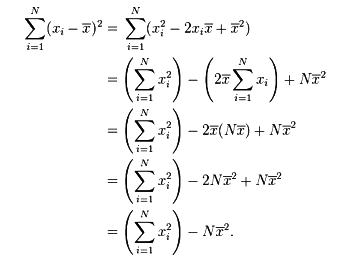# Standard Deviation

The standard deviation of a statistical population, a data set, or a probability distribution is the square root of its variance. Standard deviation is a widely used measure of the variability or dispersion, being algebraically more tractable though practically less robust than the expected deviation or average absolute deviation. A low standard deviation indicates that the data points tend to be very close to the mean, whereas high standard deviation indicates that the data are spread out over a large range of values.

In addition to expressing the variability of a population, standard deviation is commonly used to measure confidence in statistical conclusions. For example, the margin of error in polling data is determined by calculating the expected standard deviation in the results if the same poll were to be conducted multiple times. The reported margin of error is typically about twice the standard deviation – the radius of a 95% confidence interval. In science, researchers commonly report the standard deviation of experimental data, and only effects that fall far outside the range of standard deviation are considered statistically significant—normal random error or variation in the measurements is in this way distinguished from causal variation. Standard deviation is also important in finance, where the standard deviation on the rate of return on an investment is a measure of the volatility of the investment.

In finance, standard deviation is a representation of the risk associated with a given security (stocks, bonds, property, etc.), or the risk of a portfolio of securities (actively managed mutual funds, index mutual funds, or ETFs). Risk is an important factor in determining how to efficiently manage a portfolio of investments because it determines the variation in returns on the asset and/or portfolio and gives investors a mathematical basis for investment decisions (known as mean-variance optimization). The overall concept of risk is that as it increases, the expected return on the asset will increase as a result of the risk premium earned – in other words, investors should expect a higher return on an investment when said investment carries a higher level of risk, or uncertainty of that return. When evaluating investments, investors should estimate both the expected return and the uncertainty of future returns. Standard deviation provides a quantified estimate of the uncertainty of future returns.

The calculation of the sum of squared deviations can be simplified as follows: